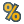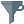#### Versions

You are viewing the RapidMiner Studio documentation for version 8.2 - Check here for latest version

#Cross Validation (Concurrency)

## Synopsis

This Operator performs a cross validation to estimate the statistical performance of a learning model.

## Description

It is mainly used to estimate how accurately a model (learned by a particular learning Operator) will perform in practice.

The Cross Validation Operator is a nested Operator. It has two subprocesses: a Training subprocess and a Testing subprocess. The Training subprocess is used for training a model. The trained model is then applied in the Testing subprocess. The performance of the model is measured during the Testing phase.

The input ExampleSet is partitioned into k subsets of equal size. Of the k subsets, a single subset is retained as the test data set (i.e. input of the Testing subprocess). The remaining k - 1 subsets are used as training data set (i.e. input of the Training subprocess). The cross validation process is then repeated k times, with each of the k subsets used exactly once as the test data. The k results from the k iterations are averaged (or otherwise combined) to produce a single estimation. The value k can be adjusted using the number of folds parameter.

The evaluation of the performance of a model on independent test sets yields a good estimation of the performance on unseen data sets. It also shows if 'overfitting' occurs. This means that the model represents the testing data very well, but it does not generalize well for new data. Thus, the performance can be much worse on test data.

## Differentiation

###Split Validation

This Operator is similar to the Cross Validation Operator but only splits the data into one training and one test set. Hence it is similar to one iteration of the cross validation.

###Split Data

This Operator splits an ExampleSet into different subsets. It can be used to manual perform a validation.

###Bootstrapping Validation

This Operator is similar to the Cross Validation Operator. Instead of splitting the input ExampleSet into different subset, the Bootstrapping Validation Operator uses bootstrapping sampling to get the training data. Bootstrapping sampling is sampling with replacement.

###Wrapper Split Validation

This Operator is similar to the Split Validation Operator. It has an additional Attribute Weighting subprocess to evaluate the attribute weighting method individually.

###Wrapper-X-Validation

This Operator is similar to the Cross Validation Operator. It has an additional Attribute Weighting subprocess to evaluate the attribute weighting method individually.

## Input

•example set (Data Table)

This input port receives an ExampleSet to apply the cross validation.

## Output

•model (Model)

This port delivers the prediction model trained on the whole ExampleSet. Please note that this port should only be connected if you really need this model because otherwise the generation will be skipped.

•performance (IOObject)

This is an expandable port. You can connect any performance vector (result of a Performance Operator) to the result port of the inner Testing subprocess. The performance output ports of the Cross Validation Operator deliver the average of the performances over the number of folds iterations.

•example set (Data Table)

This port returns the same ExampleSet which as been given as input.

•test result set (Data Table)

This port delivers only an ExampleSet if the test set results port of the inner Testing subprocess is connected. If so, the test sets are merged to one ExampleSet and delivered by this port. For example with this output port it is possible to get the labeled test sets, with the results of the Apply Model Operator.

## Parameters

• split_on_batch_attribute

If this parameter is enabled, use the Attribute with the special role 'batch' to partition the data instead of randomly splitting the data. This gives you control over the exact Examples which are used to train the model in each fold. All other split parameters are not available in this case.

Range:
• leave_one_out

If this parameter is enabled, the test set (i.e. the input of the Testing subprocess) is only one Example from the original ExampleSet. The remaining Examples are used as the training data. This is repeated such that each Example in the ExampleSet is used once as the test data. Thus it is repeated 'n' times, where 'n' is the total number of Examples in the ExampleSet. The Cross Validation can take a very long time, as the Training and Testing subprocesses are repeated as many times as the number of Example. If set to true, the number of folds parameter is not available.

Range:
• number_of_folds

This parameter specifies the number of folds (number of subsets) the ExampleSet should be divided into. Each subset has equal number of Examples. Also the number of iterations that will take place is the same as the number of folds. If the model output port is connected, the Training subprocess is repeated one more time with all Examples to build the final model.

Range:
• sampling_type

The Cross Validation Operator can use several types of sampling for building the subsets. Following options are available:

• linear_sampling: The linear sampling divides the ExampleSet into partitions without changing the order of the Examples. Subsets with consecutive Examples are created.
• shuffled_sampling: The shuffled sampling builds random subsets of the ExampleSet. Examples are chosen randomly for making subsets.
• stratified_sampling: The stratified sampling builds random subsets. It ensures that the class distribution (defined by the label Attribute) in the subsets is the same as in the whole ExampleSet. For example in the case of a binominal classification, stratified sampling builds random subsets such that each subset contains roughly the same proportions of the two values of the label Attribute.
• automatic: The automated mode uses stratified sampling per default. If it isn't applicable e.g. if the ExampleSet doesn't contain a nominal label, shuffled sampling will be used instead.
Range:
• use_local_random_seed

This parameter indicates if a local random seed should be used for randomizing Examples of a subset. Using the same value of the local random seed will produce the same subsets. Changing the value of this parameter changes the way Examples are randomized, thus subsets will have a different set of Examples. This parameter is available only if shuffled or stratified sampling is selected. It is not available for linear sampling because it requires no randomization, Examples are selected in sequence.

Range:
• local_random_seed

If the use local random seed parameter is checked this parameter determines the local random seed. The same subsets will be created every time if the same value is used.

Range:
• enable_parallel_execution

This parameter enables the parallel execution of the inner processes. Please disable the parallel execution if you run into memory problems.

Range:

## Tutorial Processes

### Why validate Models

This tutorial process shows the reason why you always have to validate a learning model on an independent data set.

The 'Sonar' data set is retrieved from the Samples folder. The Split Data Operator splits it into two different subsets (with 90 % and 10 % of the Examples). A decision tree is trained on the larger data set (which is called training data).

The decision tree is applied on both the training data and the test data and the performance is calculated for both. Below that a Cross Validation Operator is used to calculate the performance of a decision tree on the Sonar data in a more sophisticated way.

All calculated performances are delivered to the result ports of the Process:

Performance on Training data: The accuracy is relatively high with 86.63 % Performance on Test data: The accuracy is only 61.90 %. This shows that the decision tree is trained to fit the Training data well, but perform worse on the test data. This effect is called 'overfitting'. Performance from Cross Validation: The accuracy is 62.12 % +/- 9.81%. The Cross Validation not only gives us a good estimation of the performance of the model on unseen data, but also the standard deviation of this estimation. The above mentioned Perfomance on Test data falls inside this estimation, whereas the performance on the Training data is above it and is effected by 'overfitting'.### Validating Models using Cross Validation

This tutorial process shows the basic usage of the Cross Validation Operator on the 'Deals' data set from the Sample folder.

The Cross Validation Operator divides the ExampleSet into 3 subsets. The sampling type parameter is set to linear sampling, so the subsets will have consecutive Examples (check the ID Attribute). A decision tree is trained on 2 of the 3 subsets inside the Training subprocess of the Cross Validation Operator.

The performance of the decision tree is then calculated on the remaining subset in the Testing subprocess.

This is repeated 3 times, so that each subset was used one time as a test set.

The calculated performances are averaged over the three iterations and delivered to the result port of the Process. Also the decision tree, which was trained on all Examples, is delivered to the result port. The merged test sets (the test result set output port of the Cross Validation Operator) is the third result of the Process.

Play around with the parameters of the Cross Validation Operator. The number of folds parameter controls the number of subsets, the input ExampleSet is divided into. Hence it is also the number of iterations of the cross validation. The sampling type changes the way the subsets are created.

If linear sampling is used the IDs of the Examples in the subsets will be consecutive values. If shuffled sampling is used the IDs of the Examples in the subsets will be randomized. If stratified sampling is used the IDs of the Examples are also randomized, but the class distribution in the subsets will be nearly the same as in the whole 'Deals' data set.### Passing results from Training to Testing subprocess using through ports

This Process shows the usage of the through port to pass through RapidMiner Objects from the Training to the Testing subprocess of the Cross Validation Operator.

In this Process an Attribute selection is performed before a linear regression is trained. The Attribute weights are passed to the Testing subprocess. Also two different Performance Operators are used to calculate the performance of the model. Their results are connected to the expandable performance port of the Testing subprocess.

Both performances are averaged over the 10 iterations of the cross validation and are delivered to the result ports of the Process.### Using the batch Attribute to split the training data

This Process shows the usage of the split on batch attribute parameter of the Cross Validation Operator.

The Titanic Training data set is retrieved from the Samples folder and the Passenger Class Attribute is set to 'batch' role. As the split on batch attribute parameter of the Cross Validation Operator is set to true, the data set is splitted into three subsets. Each subset has only Examples of one Passenger class.

In the Training subprocess, 2 of the subsets are used to train the decision tree. In the Testing subprocess, the remaining subset is used to test the decision tree.

Thus the decision tree is trained on all passengers from two Passenger Classes and tested on the remaining class. The performances of all three combinations are averaged and delivered to the result port of the Process.